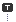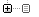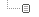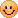Every unexpected event is a path to learning for you.

카테고리분류 전체보기 (2307)Unity3D (564)Programming (470)Unreal (4)Gamebryo (56)Tip & Tech (182)협업 (34)3DS Max (3)Game (12)Utility (114)Etc (92)Portfolio (19)Subject (90)iOS,OSX (37)Android (12)Linux (5)잉여 프로젝트 (2)게임이야기 (1)Memories (19)Interest (37)Thinking (36)한글 (26)PaperCraft (5)Animation (408)Wallpaper (2)재테크 (19)Exercise (3)나만의 맛집 (2)냥이 (9)육아 (5)
Total1,331,749
Today22
Yesterday113달력

« » 2019.10
1 2 3 4 5
6 7 8 9 10 11 12
13 14 15 16 17 18 19
20 21 22 23 24 25 26
27 28 29 30 31

'operator'에 해당되는 글 1건

1. 2011.12.05 Operator Overloading in C# (2)

Programming/C# / 2011.12.05 11:35

Description

The Source code below shows how to use OperatorOverloading in C#. Operator Overloading is pretty useful concept derived from C++ by C#.For eg.

We can have class Matrix which hold 2D array of elements.C# add doest not Allows us to do Addition, Subtraction of matrices using the +,- operator rather We use to write Methods AddMatrix(),SubMatrix() with the use of Operator.

Overloading we make the user to code it:

M3=M1+M2 where M1 and M2 are matrix objects.

1. While OverLoading a Operator always give public static access specifiers.

2. Operator Overloading should return a CLS type and not void.

3. Atleast one operand to the operator must be of type UDC [User Defined Class] because we cannot overload a operator with two int operands and perform subtraction for + operator which C# does not allow.

4. Relational Operators are overloaded in Pairs only.ie if == is overloaded then So !=.

5. Same Operator can be overloaded with different function signature for eg.
public static Matrix operator +(Matrix m1,int[,] m2)

we can also one more method like this:
public static Matrix operator + (Matrix m1,Matrix m2)

Please find to the code which uses overloading of almost all operators.

Source Code:

// Source Code starts
using System;
class Square
{
private double Side;
//public Constructor if int is passed convert to double and assign to Side
public Square(int s)
{
Console.WriteLine(".ctor with int argument");
Side=(
double)s;

//OverLoaded constructor with double argument
public Square(double s)
{
Console.WriteLine(".ctor with double argument");
Side=s;

//override ToString() method of object class.
public override string ToString()
{
Console.WriteLine("Override object class's string");
return this.Side.ToString();

//Overloading + operator to add 2 square objects and return new square object
public static Square operator + (Square x,Square y)
{
return new Square(x.Side+y.Side);

//Overloading + operator to add square objects with double side and return new square object
public static Square operator + (Square x,double y)
{
return new Square(x.Side+y);

//Overloading + operator to add square objects with int side and return new square object
//This is not necessary since C# automatically calls +(Square,double)
public static Square operator + (Square x,int y)
{
return x +(double)y;

public static implicit operator Square(double s)
{
return new Square(s);

public static implicit operator Square(int s)
{
return new Square((double)s);

public static bool operator ==(Square x,Square y)
{
return x.Side==y.Side;
}
public static bool operator !=(Square x,Square y)
{
return !(x==y); //This will call to operator == simple way to implement !=

public override bool Equals(object o)
{
return this==(Square)o;

public override int GetHashCode()
{
return (int)Side;

public static bool operator >(Square x,Square y)
{
return x.Side>y.Side;

public static bool operator <(Square x,Square y)
{
return x.Side<y.Side;

public static bool operator <=(Square x,Square y)
{
return (x<y) || (x==y); //Calls to operator == and <

public static bool operator >=(Square x,Square y)
{
return (x>y) || (x==y); //Calls to operator == and >

public double Area
{
get
{
return 2*Side;
}

public static void Main()
{
Square s1=
new Square(10);
Square s2=
new Square(20);
Square s3=s1+s2;
// This will call operator + (Square,Square)
Console.WriteLine(s3);
Console.WriteLine(s3+15);
// This will call operator + (Square,int) and then ToString()
Console.WriteLine(s3+1.5);// This will call operator + (Square,double) and then ToString()
s3=10; // This will call operator Square(int)
Console.WriteLine(s3);
Square s4=10;
Console.WriteLine(s1==s4);
//Calls == operator
Console.WriteLine(s1!=s4); //Calls != operator
Console.WriteLine(s1>s2); //Calls > operator
Console.WriteLine(s1<=s4); //Calls <= operator
}
}
// Source Code End

'Programming > C#' 카테고리의 다른 글

 Convert String To Enum Instance  (0) 2011.12.16 2011.12.16 2011.12.05 2011.11.29 2011.11.29 2011.11.29
TAG

댓글을 달아 주세요

1. 2013.01.05 08:28 C. 탄소

좋은 정보 감사합니다. C#에서 연산자 오버로딩을 할 때 static을 사용하는 건,
연산자를 오버로딩할 때 인자로 적어도 하나 이상의 UDC 인스턴스를 넣기 위함이었을지도 모르겠다는 생각이 드네요..

• 2013.01.08 11:33 신고 blueasa

도움이 됐다니 즐겁네요.
요즘 날씨가 너무 춥습니다. 감기 조심하세요.